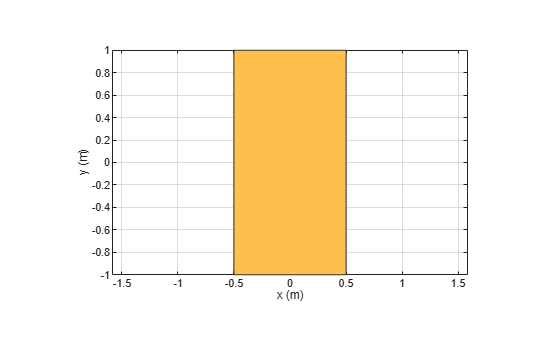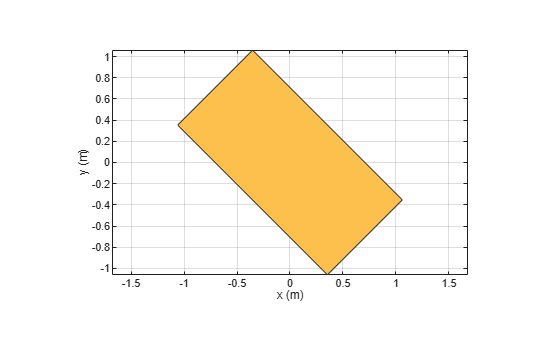# rotate

Rotate shape about axis and angle

## Syntax

``rotate(shape,angle,axis1,axis2)``
``c = rotate(shape,angle,axis1,axis2)``

## Description

example

````rotate(shape,angle,axis1,axis2)` rotate shape about an axes object and angle.```
````c = rotate(shape,angle,axis1,axis2)` rotate shape about an axes object and angle.```

## Examples

collapse all

Create a rectangle shape.

```r = antenna.Rectangle; show(r) axis equal```Rotate the rectangle at 45 degrees about the Z-axis.

`r1 = rotate(r,45,[0 0 0],[0 0 1])`
```r1 = Rectangle with properties: Name: 'myrectangle' Center: [0 0] Length: 1 Width: 2 NumPoints: 2 ```
`show(r1)`## Input Arguments

collapse all

Shape created using custom elements and shape objects of Antenna Toolbox, specified as an object.

Example: `area(rectangle)` where rectangle is created using `antenna.Rectangle` object.

Axis of rotation, specified as two unique three-element vectors of Cartesian coordinates in meters.

Example: `rotate(rectangle,45,[0 0 0], [0 0 1])` where rectangle is created using `antenna.Rectangle` object.

Data Types: `double`

Angle of rotation, specified as a scalar in degrees.

Example: `rotate(rectangle,45,[0 0 1], [0 0 0])` rotates the rectangle around X-axis by 45 degrees.

Data Types: `double`

## Version History

Introduced in R2017a